JMSLTM Numerical Library 7.2.0
com.imsl.stat

## Class HoltWintersExponentialSmoothing

• All Implemented Interfaces:
Serializable, Cloneable

```public class HoltWintersExponentialSmoothing
extends Object
implements Serializable, Cloneable```
Calculates parameters and forecasts using the Holt-Winters Multiplicative or Additive forecasting method for seasonal data.

`HoltWintersExponentialSmoothing` performs the Holt-Winters forecasting method to an equally spaced time series,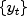where N = `nobs` and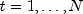(or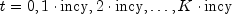where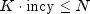and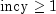). The Holt-Winters procedure fits three component sequences known as the level, the trend, and the seasonal sequence. There are two formulations, depending on whether the seasonal component of the time series is thought to be additive or multiplicative. The seasonal component depends on the length of the season, `nseason` = s, where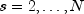.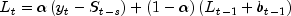the level sequence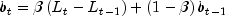the trend sequence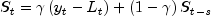the seasonal sequence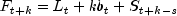the forecast sequence

### Holt-Winters Multiplicative Model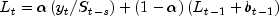the level sequencethe trend sequence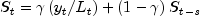the seasonal sequencethe forecast sequence

Note that without a seasonal component, both the additive and multiplicative formulations reduce to the same methods. (The seasonal sequence is set to 1 for the multiplicative model, and identically 0 for the additive model.)

### Default Starting Values

Initial values are required for these sequences. The software allows the user code to define these initial values (see `setInitialValues` method). If they are not provided, then the first two seasons of data are used: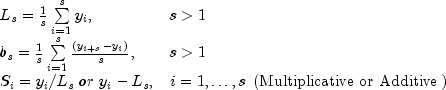The smoothing parameters (,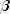,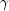) are each in the interval [0,1] and can be specified by the user (see `setParameters` method), or automatically set by minimizing the within sample one-step ahead mean squared forecast error. Note that this objective function is not necessarily convex and solutions will depend on starting values. See Chatfield and Yar(1988) for further discussion. Starting values for (,,) are obtained by minimizing the mean squared error over `nsamples` bootstrap samples of the time series. Experiments suggest that this approach helps prevent poor starting values. Note, that solutions may vary for different settings of the number of random samples, `nsamples`.

The return value of the `compute` method is an (N + 1) by 3 = (`nobs` + 1) by 3 matrix containing the smoothing parameter values on the first row, followed by the calculated level, trend, and seasonal sequences. When N = `nobs` and s = `nseason`, the format of the return value is as follows:

Series Storage
Row Value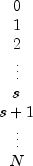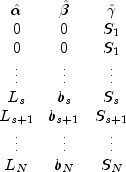If one of the nonseasonal options is specifed, the return value will be (`nobs` + 1) by 2 or (`nobs` + 1) by 1 accordingly.

The variance-covariance matrix for the parameters (,,) is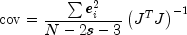where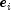is the one-step-ahead forecast error, and J is the Jacobian matrix of the series using the forecast model and calculating forecasts one step ahead of each datum. Prediction intervals are calculated following Chatfield and Yar (1991).

Example, Serialized Form
• ### Constructor Summary

Constructors
Constructor and Description
```HoltWintersExponentialSmoothing(int nseason, double[] y)```
Constructor for `HoltWintersExponentialSmoothing`.
• ### Method Summary

Methods
Modifier and Type Method and Description
`double[][]` `compute()`
Computes the values of the smoothing parameters.
`double[][]` `getForecasts()`
Returns the forecasts past the series data.
`double[]` `getSmoothedSeries()`
Returns the fitted series values.
`double` `getSumOfSquares()`
Returns the sum of squares of the one step ahead forecast errors.
`double[][]` `getVarCovarMatrix()`
Returns the variance-covariance matrix of the smoothing parameters estimated by minimizing the mean squared forecast error.
`void` `setAdditive()`
Specifies the use of the Additive time series model.
`void` `setConfidence(double confid)`
Sets the confidence level to use in the calculation of the prediction intervals.
`void` `setInitialValues(double[][] sequences)`
Sets the initial values for the level, trend, and seasonal component sequences.
`void` `setLowerBounds(double[] xlb)`
Sets the lower bounds for each of the smoothing parameters, (,,).
`void` `setNonseasonalConstant()`
Remove the trend and the seasonal components and fit only the level component.
`void` `setNonseasonalTrend()`
Remove the seasonal component and fit only the level and trend components.
`void` `setNumberEval(int nsamples)`
Sets the number of evaluations of the residual norm that are sampled to obtain starting values for the smoothing parameters, (,,).
`void` `setNumberForecasts(int nforecasts)`
Sets the number of forecasts desired past the series data.
`void` `setNumberOfObservations(int nobs)`
Sets the number of equally spaced series values.
`void` `setParameters(double[] params)`
Sets the values of the smoothing parameters for the level (), the trend (), and the seasonal () component sequences.
`void` `setSeriesIncrement(int incy)`
Sets the constant stride through the series data `y`.
`void` `setUpperBounds(double[] xub)`
Sets the upper bounds for each of the smoothing parameters, (,,).
• ### Methods inherited from class java.lang.Object

`clone, equals, finalize, getClass, hashCode, notify, notifyAll, toString, wait, wait, wait`
• ### Constructor Detail

• #### HoltWintersExponentialSmoothing

```public HoltWintersExponentialSmoothing(int nseason,
double[] y)```
Constructor for `HoltWintersExponentialSmoothing`.
Parameters:
`nseason` - an `int` scalar containing the number of time points in a season, or the length of the season. The class requires that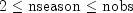unless one of the non-seasonal methods is used, in which case `nseason` is ignored. See the `setNonseasonalTrend` and `setNonseasonalConstant` methods for details.
`y` - a `double` array containing the values of the time series.
• ### Method Detail

• #### compute

`public final double[][] compute()`
Computes the values of the smoothing parameters.
Returns:
an (`nobs` + 1) by 3 `double` matrix containing the values of the smoothing parameters followed by the level, trend, and seasonal component sequences. Note that if the `setNonseasonalTrend` method is used, the matrix is of dimension (`nobs` + 1) by 2 and if the `setNonseasonalConstant` method is used, it is (`nobs` + 1) by 1.
• #### getForecasts

`public double[][] getForecasts()`
Returns the forecasts past the series data.
Returns:
an `nforecasts` by 1 `double` matrix containing the forecasts past the series data. The value of the i-th row is the forecast (i + 1) steps past the series data. If `setConfidence` is used, the matrix will be of dimension `nforecasts` by 3 and the value of the i-th row is the forecast (i+1) steps ahead followed by the prediction interval lower and upper bounds.
• #### getSmoothedSeries

`public double[] getSmoothedSeries()`
Returns the fitted series values.
Returns:
a `double` array containing the fitted series values.
• #### getSumOfSquares

`public double getSumOfSquares()`
Returns the sum of squares of the one step ahead forecast errors.
Returns:
a `double` scalar containing the sum of squares of the one step ahead forecast errors.
• #### getVarCovarMatrix

`public double[][] getVarCovarMatrix()`
Returns the variance-covariance matrix of the smoothing parameters estimated by minimizing the mean squared forecast error. The matrix is 3 by 3 unless the `setNonseasonalTrend` method is used, in which case it is 2 by 2, or unless the `setNonseasonalConstant` method is used, in which case it is 1 by 1. This method cannot be used together with the `setParameters` method. Note that the `compute` method must be invoked before invoking this method. Otherwise, the method throws a `NullPointerException` exception.
Returns:
a `double` matrix containing the variance-covariance matrix of the smoothing parameters estimated by minimizing the mean squared forecast error.

`public void setAdditive()`
Specifies the use of the Additive time series model. The Multiplicative model is the default.
• #### setConfidence

`public void setConfidence(double confid)`
Sets the confidence level to use in the calculation of the prediction intervals.
Parameters:
`confid` - a `double` scalar containing the confidence level to use in the calculation of the prediction intervals. If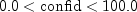, prediction intervals are provided for each forecast.

Default: Prediction intervals are not provided.

• #### setInitialValues

`public void setInitialValues(double[][] sequences)`
Sets the initial values for the level, trend, and seasonal component sequences.
Parameters:
`sequences` - an (`nobs` + 1) by 3 `double` matrix containing the initial values for the level , trend, and seasonal component sequences. The values must be stored in rows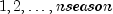. Rows 0 and `nseason` + 1 to `nobs` are ignored on input. `sequences.length` should be equal to 3 unless the `setNonseasonalTrend` method is used, in which case it is 2 containing initial values for level () and the trend (). Likewise, if the ``` setNonseasonalConstant``` method is used, ``` sequences.length``` is 1 and contains the initial values for the level parameter () only.

Default: Initial values are computed by the function.

• #### setLowerBounds

`public void setLowerBounds(double[] xlb)`
Sets the lower bounds for each of the smoothing parameters, (,,).
Parameters:
`xlb` - a `double` array containing the lower bounds for each of the smoothing parameters, (,,). Note that the lower bounds must be in the interval [0,1], inclusive. The array is ignored if the `setParameters` method is used. The array should be length 3 unless the `setNonseasonalTrend` method is used, in which case it is of length 2 containing lower bounds for level () and the trend () components. Likewise, if the `setNonseasonalConstant` method is used, `xlb` is of length 1 and contains the lower bound for the level parameter () component only.

Default: Lower bounds are all 0.

• #### setNonseasonalConstant

`public void setNonseasonalConstant()`
Remove the trend and the seasonal components and fit only the level component. If used, the models involve only the level () parameter. The method is simple exponential smoothing.

Default: The method includes all three components.

• #### setNonseasonalTrend

`public void setNonseasonalTrend()`
Remove the seasonal component and fit only the level and trend components. If used, the models involve only the level () and trend () parameters. The method is equivalent to double exponential smoothing.

Default: The method includes all three components.

• #### setNumberEval

`public void setNumberEval(int nsamples)`
Sets the number of evaluations of the residual norm that are sampled to obtain starting values for the smoothing parameters, (,,).
Parameters:
`nsamples` - an `int` scalar containing the number of evaluations.

Default: `nsamples` = `nobs`.

• #### setNumberForecasts

`public void setNumberForecasts(int nforecasts)`
Sets the number of forecasts desired past the series data.
Parameters:
`nforecasts` - an `int` scalar containing the number of forecasts desired past the series data.

Default: No forecasts are computed past the series data.

• #### setNumberOfObservations

`public void setNumberOfObservations(int nobs)`
Sets the number of equally spaced series values.
Parameters:
`nobs` - an `int` scalar containing the number of equally spaced series values.

Default: `nobs` = `y.length`.

• #### setParameters

`public void setParameters(double[] params)`
Sets the values of the smoothing parameters for the level (), the trend (), and the seasonal () component sequences.
Parameters:
`params` - a `double` array containing the values of the smoothing parameters for the level (), the trend (), and the seasonal () component sequences. The array should be length 3 unless the `setNonseasonalTrend` method is used, in which case it is of length 2 containing values for level () and the trend () components. Likewise, if the `setNonseasonalConstant` method is used, `params` is of length 1 and contains the value for the level parameter () component only.

Default: Parameter values are selected by minimizing the mean squared one step ahead forecast error.

• #### setSeriesIncrement

`public void setSeriesIncrement(int incy)`
Sets the constant stride through the series data `y`.
Parameters:
`incy` - an `int` scalar containing the constant stride through the series data `y`. ```y.length ``` must be at least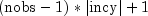. When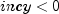, the series is incremented in reverse order beginning at index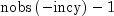.

Default: `incy` = 1.

• #### setUpperBounds

`public void setUpperBounds(double[] xub)`
Sets the upper bounds for each of the smoothing parameters, (,,).
Parameters:
`xub` - a `double` array containing the upper bounds for each of the smoothing parameters, (,,). Note that the upper bounds must be in the interval [0,1], inclusive. The array is ignored if the `setParameters` method is used. The array should be length 3 unless the `setNonseasonalTrend` method is used, in which case it is of length 2 containing upper bounds for level () and the trend () components. Likewise, if the `setNonseasonalConstant` method is used, `xub` is of length 1 and contains the upper bound for the level parameter () component only.

Default: Upper bounds are all 1.

JMSLTM Numerical Library 7.2.0